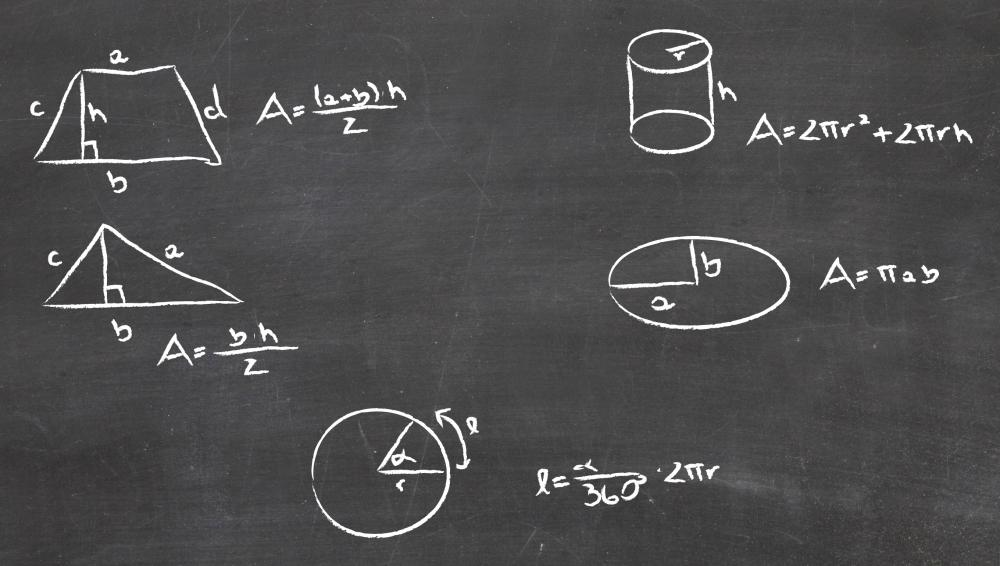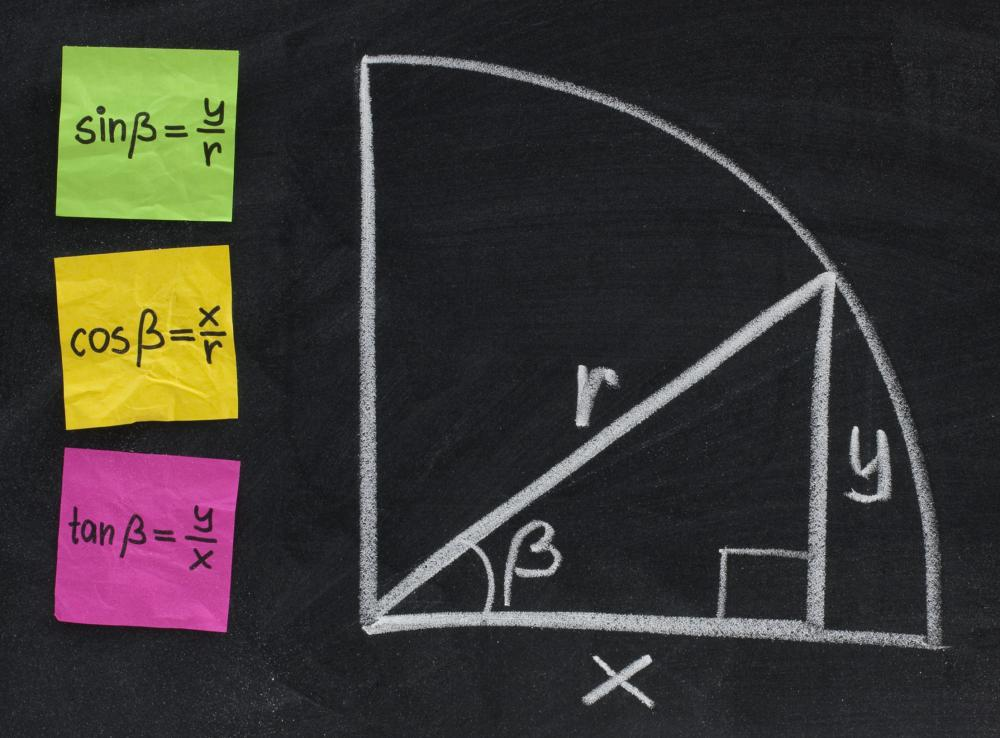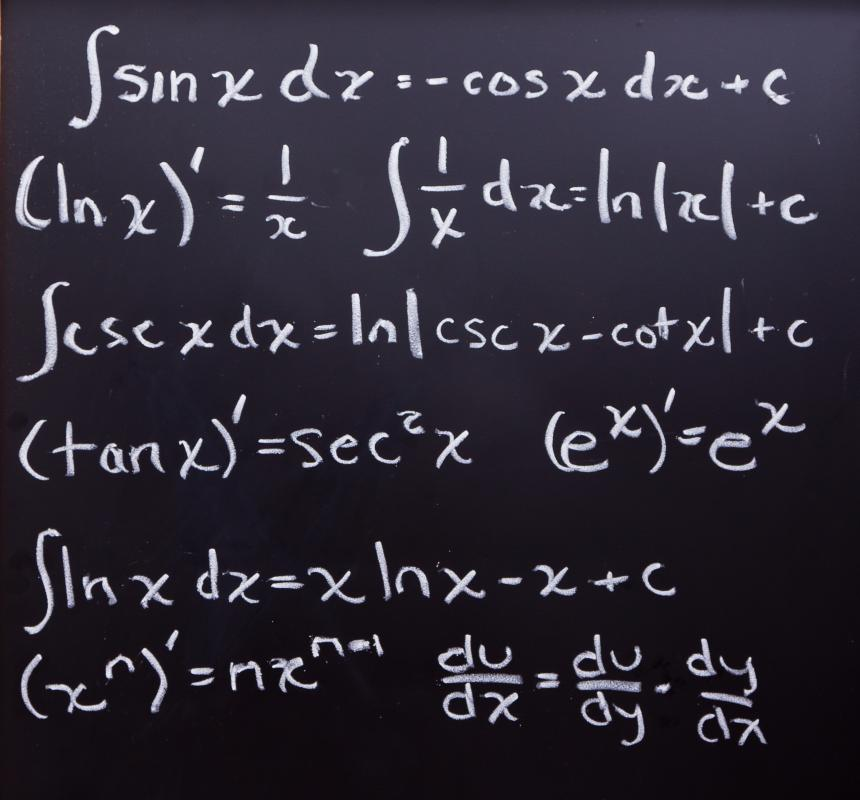# What are the Different Disciplines of Mathematics?

Tricia Christensen
Tricia ChristensenAlgebra is a discipline of mathematics wherein a student must find an unknown variable by solving an equation.

Mathematics includes many disciplines that have evolved throughout history. Simple examples include addition and subtraction, while extremely complex forms include chaos and games theories. Traditionally, however, the high school and early college years focus on the following disciplines.

Algebra is the gateway to most mathematics studies. Students may study algebra in 8th, 9th, or 10th grade, depending on their skills and school requirements. Normally, first year algebra concerns itself with the study of equations to find unknown elements. Students learn how to solve equations for two to three variables, depending on the class.Geometry is the discipline of measuring objects and often utilizes algebra to solve for unknown variables of a geometric principle.

Generally, the study of algebra is followed by the study of geometry, which is usually taken after a first year algebra course. A second year algebra course includes geometric principals. In colleges, the study of algebra is often combined with the study of geometry, and students do not take a separate course.

Most teachers start teaching basic algebraic and geometric concepts long before students actually take those classes. In many cases, students are now doing one and two-step equations solving for a variable by third or fourth grade. The familiarity with solving equations is thought to help prepare the student for working with multiple variables in first year algebra.Trigonometry is the discipline concerned with angles and shapes, and is frequently used in the field of astronomy.

Students also often learn basic formulas for measuring objects, like triangles, squares, and circles, by fifth or sixth grade. This early preparation in mathematics rarely questions the “why” of a formula, but prepares the students to ask it. This question will be answered by the proofs and theorems that dictate and explain why formulas for obtaining measurements in shapes actually work.Calculus is an advanced mathematical discipline that is a prerequisite for college-level science courses, such as physics.

Some students end their mathematics education with second year algebra. Many, however, go on to study trigonometry, a branch that deals with the principles of angles and shapes. Some feel that trigonometry is advanced geometry, while others argue it is a completely separate area of study. It has broad applications, but one most familiar to people is its use in astronomy to measure the distance of stars and planets from each other in a process called triangulation.

After trigonometry, students often study calculus, which is developed from advanced algebra and geometry. In many colleges, students can study either calculus or trigonometry as a final mathematics course. Calculus is actually two different branches: differential and integral. Differential calculus concerns itself with equations measuring things like distance and velocity. Integral calculus evaluates geometry with attention to real world applications, like how time or temperature might affect an equation.

Both forms of calculus are essential to understanding applications in major sciences like physics. In fact, in most colleges, one must at least be eligible to take calculus courses prior to enrolling in physics courses. Some advanced science courses require that one has already taken calculus, since calculus equations are fundamental to understanding the more complex aspects of a science.

Another branch of mathematics is the field of statistics and probability. Those studying economics or accounting must usually take a course in one or the other — or in both — to meet graduation requirements for college. Beyond these branches, there are many other sub-fields that become very specific in their applications. Math majors will pursue these courses in order to earn advanced degrees.Tricia Christensen

Tricia has a Literature degree from Sonoma State University and has been a frequent contributor for many years. She is especially passionate about reading and writing, although her other interests include medicine, art, film, history, politics, ethics, and religion. Tricia lives in Northern California and is currently working on her first novel.Tricia Christensen

Tricia has a Literature degree from Sonoma State University and has been a frequent contributor for many years. She is especially passionate about reading and writing, although her other interests include medicine, art, film, history, politics, ethics, and religion. Tricia lives in Northern California and is currently working on her first novel.

## You might also LikeEmilski

@kentuckycat - That's funny, because I am the complete opposite. I love doing math, but I'm not very good at it. It sort of depends on the problems, though.

When I was in high school, I took calculus and was very good at it. I was even at the point where I could do a lot of problems in my head. Once I got to college, though, a lot of my math classes were in statistics.

There is something inherent about statistics that I don't get. I guess it is just the way my brain works. The way experiments are set up and what analyses they need always makes me have to stop and think.

kentuckycat

I was never aware that there were two different areas of calculus. I took it in high school, but we never had it broken down like that. Now that I think about it, though, we definitely had a beginning section talking about integrals and then moved into differential problems.

I always had an odd relationship with math classes. I was always very good at math, but I hated doing it. I always got great grades and was at the top of the class, but doing the problems made me miserable. Everyone said I should go to school for engineering, but I think I would have gone crazy after the first week!

matthewc23

@anon164182 - I would also add that one of the reasons universities offer those types of advanced classes and not things like beginning algebra and trigonometry is because people who actually need those classes to advance in college have already taken them.

I find it hard to believe that someone studying astronomy or physics didn't take trigonometry and calculus in high school. By the time they get to college, they are ready for those classes like differential equations and nonlinear algebra.

If you look at the curricula of liberal arts or community colleges, they will often offer lower level math courses, because their students may just need a basic understanding of math to advance.

Izzy78

@anon164182 - I don't see where else trigonometry would belong if it isn't mathematics. Everything revolves around numbers and equations. I looks at trigonometry as the link between algebra and calculus.

You need algebra to be able to figure out trigonometric concepts like missing angles and everything else to do with triangles.

To be able to understand basic calculus concepts, you need to first understand the things you learned in trigonometry like the various functions. Without knowing how triangles work, you could never use the unit circle in calculus.

anon241756

A simple pendulum has time period t on surface of earth. What will be its time period at a height r/2 from surface of earth.(Where r is radius of earth).

anon228489

to elfi64: I hope your post was meant as a joke.

anon164182

Unfortunately, this article was clearly not written by a mathematician. As a math professor, I would not consider trigonometry a discipline of mathematics, for example. As a mathematics major at a university, you would see calculus and perhaps probability and statistics, depending on your focus, but primarily you would take classes in areas that are not even mentioned in this article: linear algebra, abstract algebra, differential equations, real and complex analysis, logic, topology, algebraic geometry. Those areas are where the new developments in mathematics are happening, as well, rather than in high school geometry or calculus.

anon100819

The few core concepts that ground all math were grasped long ago, but the more abstruse elements have come only recently, but they have come in spades. Look up advancements in mathematics and you will see. Like me, you probably won't have a clue what you're looking at, but you'll be impressed nonetheless.

elfi64

It seems that mathematics is a science that is rather dormant. What was figured out was done in the past. There are rare new developments in the field of mathematics.